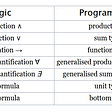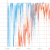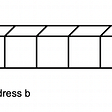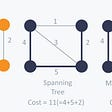# Selection, Insertion, and Merge Sort

In this post, we’re going to explore a few basic sorting algorithms.

# Selection Sort

This is the simplest and most naive approach to sort an array. As you iterate an array, you select the smallest among the remaining elements and swap them.

`for (i=0; i<N; i++):  min = i  for (j=i+1; j<n; j++):    if arr[j] < arr[min]:      min = j  swap(i, min)`

# Insertion Sort

`for (i=1; i<N; i++):  for (j=i; i>=0; j--):    if (arr[j] < arr[j-1]):      swap(j, j-1)    else:  // stop cuz it's already sorted      break`

## Time Complexity

In the best case,
It’s already sorted, so we don’t need to iterate backwards every iteration.
N-1 compares, 0 exchanges

In the worst case,
It’s sorted in reverse order.
N²/2 compares, N²/2 exchanges

On average,
N²/4 compares, N²/4 exchanges

# Merge Sort

John Von Neumann suggested Merge Sort, which is based on Divide and Conquer paradigm. The key idea is to keep dividing the array into two, thus making it easier to sort them, and merging them into a complete sorted array.

Here’s a Python code below.

`def mergeSort(array):    if len(array) > 1:        mid = len(array) // 2                I = array[:mid]        J = array[mid:]                mergeSort(I)        mergeSort(J)                i = k = j = 0                while i < len(I) or j < len(J):            if i == len(I):                array[k:k+len(J)-j] = J[j:len(J)]                break                        if j == len(J):                array[k:k+len(I)-i] = I[i:len(I)]                break                            if I[i] <= J[j]:                array[k] = I[i]                i += 1                            else:                array[k] = J[j]                j += 1                            k += 1`

## Time Complexity

It’s an optimal compare-based algorithm with its time complexity O(NlogN) being the lower bound. For memory, it uses an extra space proportional to N, though.

# In-place & Stable

## In-place

An algorithm is said to be in-place if it uses less than C log N extra memory. A selection and insertion algorithm is so.

## Stable

An algorithm is stable if it preserves the order of records with equal keys.

In the example above, a stable algorithm produces the upper table in the far right. You can observe that the records are ordered by surname as well.

A merge sort and insertion sort are stable. If you think about it, you only swap two local elements in an insertion sort. In a merge sort, locally, you’re sorting two sorted arrays.

A selection sort is not stable. You can’t guarantee that it preserves the orders.

--

--

--

Hello, world.

## Bring your game to AAA status through Post Processing!## Bitcoin, Supersonic Flight and Christmas Lights## From logic and math to code. For dummies. Part III. Dependent types## Working with Arrays in Go(golang)## He Always Asks Why. Meet Andrzej — a Senior Android Developer## DevConf: sketchnotes and a workshop## Visual Studio Code: A Newbie’s Guide to Personalizing your Workspace and Why it Might Matter## The Magic Behind Service Workers## Abstract Data Types and Data Structures## LeetCode — Spiral Matrix## Minimum Spanning Tree & Prim’s Algorithm